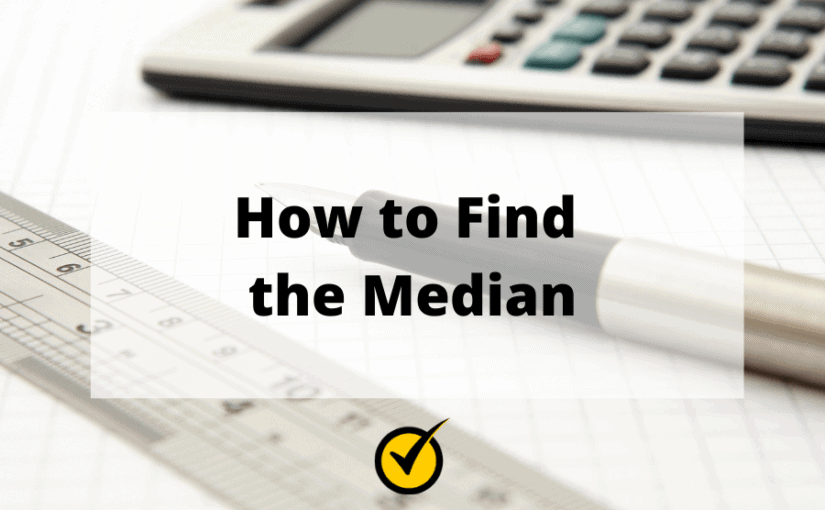# How to Find the Median

The median is the middle number in a range of numbers.

Let’s say that you’re helping your child with their homework, and you’re given a list of numbers with the directions to find the median.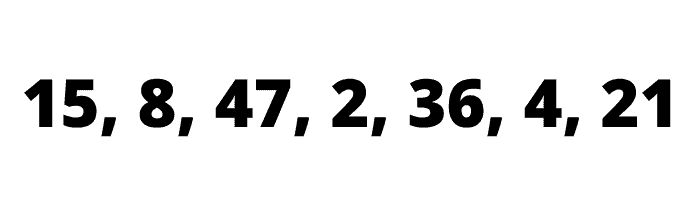To find the median of these numbers, you would first put the numbers in order from lowest to highest: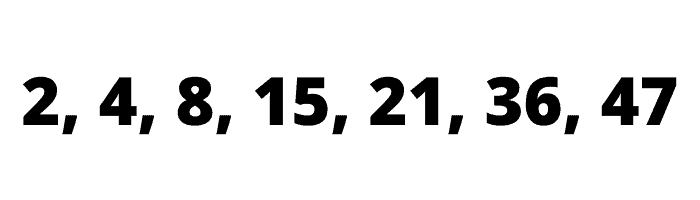Then look for the number that falls in the middle.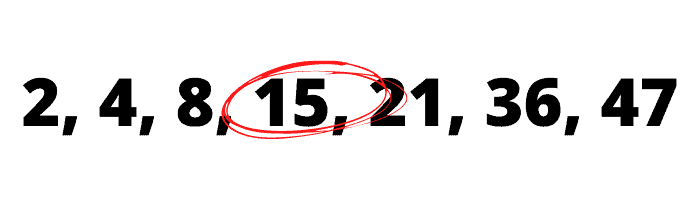The number 15 falls in the middle. There are three numbers to the left of 15 and three numbers to the right of 15, making 15 the middle number.

When there is an odd amount of numbers in a list, it’s easy to find the middle number.

However, it’s a little more difficult to find the middle number when you’re dealing with a list containing an even amount of numbers.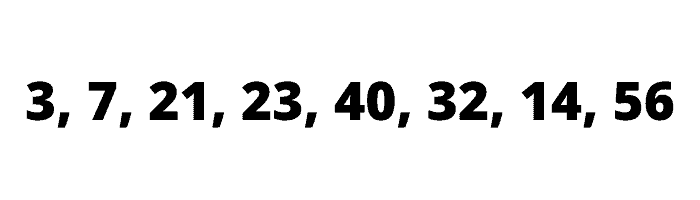First, you’ll need to put the numbers in order from lowest to highest.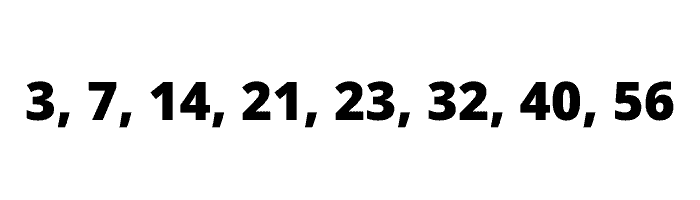There are 8 numbers in the list, so there is not just one middle number, but two.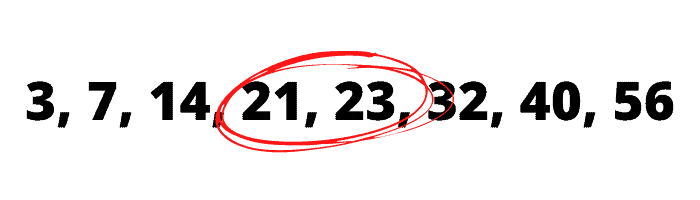To find the value halfway between these two numbers, we add them together first: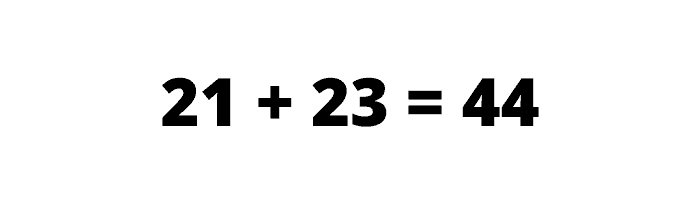Then we divide the answer by 2:So the median of this list of numbers is 22.